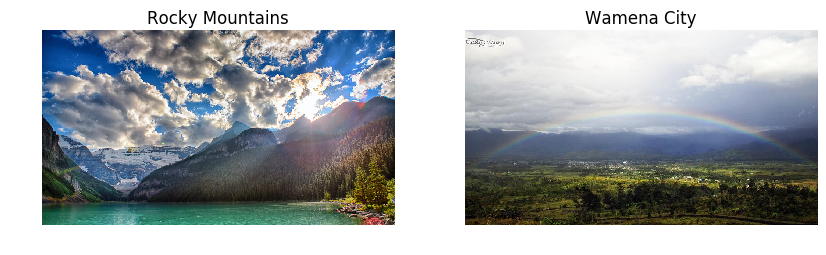# Plain Vanilla Python Convolution Filter

Convolution_ImageProcessing

# Applying Convolution Filters in Image Processing¶

In other blogs I used built-in Sobel, Canny, etc. functions to apply filters. In this one we build convolution function in Python and apply filters directly.

In :
import numpy as np
import matplotlib.pyplot as plt

# Make sure plot is embeded
%matplotlib inline

plt.rcParams['figure.figsize'] = (10.0, 8.0) # set default size of plots
plt.rcParams['image.interpolation'] = 'nearest'
plt.rcParams['image.cmap'] = 'gray'


In :
# Load original image
d0 = img.shape - img2.shape
d1 = img2.shape - img.shape
img = img[d0/2:-d0/2, :, :]
img2 = img2[:, d1/2:-d1/2,:]
plt.subplot(1,2,1)
plt.imshow(img)
plt.axis('off')
plt.title('Rocky Mountains')
print "Image size is: ", img.shape
#If run time is too long, make image smaller
#small_img = imresize(img, (64, 96), interp='bicubic')
#plt.imshow(small_img)
plt.subplot(1,2,2)

plt.axis('off')
plt.title('Wamena City')
plt.imshow(img2)
plt.show()
x = np.zeros((2, img.shape, img.shape, img.shape))
x[0, :, :, :] = imresize(img, (img.shape, img.shape)).transpose((2, 0, 1))
x[1, :, :, :] = imresize(img2, (img2.shape, img2.shape)).transpose((2, 0, 1))

Image size is:  (532, 960, 3)In :
# Define Convolutional Operations, both forward and backward functions
# Based on cs231n project #2

def conv_forward(x, w, b, conv_param):
"""
A naive implementation of the forward pass for a convolution function.
Apply multiple filters (w) to input image at the same time.

The input consists of N data points, each with C channels, height H and width
W. We convolve each input with filter, where each filter spans
all C channels and has height HH and width HH.

Input:
- x: Input data of shape (N, C, H, W); C: Num of Channels, H: Height, W: width
- w: Filter weights of shape (F, C, HH, WW); F: number of filters, HH: filter Height, WW: width
- b: Biases, of shape (F,)
- conv_param: A dictionary with the following keys:
- 'stride': The number of pixels between adjacent receptive fields in the
horizontal and vertical directions.
- 'pad': The number of pixels that will be used to zero-pad the input.

Returns a tuple of:
- out: Output data, of shape (N, F, H', W') where H' and W' are given by
H' = 1 + (H + 2 * pad - HH) / stride
W' = 1 + (W + 2 * pad - WW) / stride
- cache: (x, w, b, conv_param)
"""
out = None
N, C, H, W = x.shape
F, _, HH, WW = w.shape
stride = conv_param['stride']
OH = 1 + (H + 2 * pad - HH) / stride
OW = 1 + (W + 2 * pad - WW) / stride
out = np.zeros((N, F, OH, OW))
for t in xrange(N):
input = x_aug[t,:,:,:]
for f in xrange(F):
for i in xrange(OH):
for j in xrange(OW):
x_win = input[:, i*stride:i*stride+HH, j*stride:j*stride+WW]
filter = w[f,:,:,:]
out[t, f, i, j] = np.sum(x_win * filter) + b[f]
cache = (x, w, b, conv_param)
return out, cache

In :
# Set up a convolutional weights holding 2 filters, each 3x3
w = np.zeros((2, 3, 3, 3))

# The first filter converts the image to grayscale.
# Set up the red, green, and blue channels of the filter.
w[0, 0, :, :] = [[0, 0, 0], [0, 0.3, 0], [0, 0, 0]]
w[0, 1, :, :] = [[0, 0, 0], [0, 0.6, 0], [0, 0, 0]]
w[0, 2, :, :] = [[0, 0, 0], [0, 0.1, 0], [0, 0, 0]]

# Second filter detects horizontal edges in the blue channels.
w[1, 1, :, :] = [[1, 2, 1], [0, 0, 0], [-1, -2, -1]]

# Vector of biases. We don't need any bias for the grayscale
# filter, but for the edge detection filter we want to add 128
# to each output so that nothing is negative.
b = np.array([0, 128])

# Compute the result of convolving each input in x with each filter in w,
# offsetting by b, and storing the results in out.
out, _ = conv_forward(x, w, b, {'stride': 1, 'pad': 1})

def imshow_noax(img, normalize=True):
""" Tiny helper to show images as uint8 and remove axis labels """
if normalize:
img_max, img_min = np.max(img), np.min(img)
img = 255.0 * (img - img_min) / (img_max - img_min)
plt.imshow(img.astype('uint8'))
plt.gca().axis('off')

# Show the original images and the results of the conv operation
plt.subplot(2, 3, 1)
imshow_noax(img, normalize=False)
plt.title('Original image')
plt.subplot(2, 3, 2)
imshow_noax(out[0, 0])
plt.title('Grayscale')
plt.subplot(2, 3, 3)
imshow_noax(out[0, 1])
plt.title('Edges')
plt.subplot(2, 3, 4)
imshow_noax(img2, normalize=False)
plt.subplot(2, 3, 5)
imshow_noax(out[1, 0])
plt.subplot(2, 3, 6)
imshow_noax(out[1, 1])
plt.show()